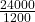Question

1. A 5.00 kg bowling ball is rolling at 6.00 m/s. What is the bowling balls momentum.
2. A car with mass 1200. kg has momentum 24 000 kg m/s. How fast is the car traveling.

Example: Your physics teacher is walking at 2.00 m/s. His momentum is 300. kg m/s. What is his mass?
v = 2.00 m/s ; p = 300. kgm/s ; m = ?
p = m*v ; m = p / v ; m = (300. kgm/s) / (2.00 m/s) ; m = 150. kg

in progress 0
5 months 2021-08-11T10:10:14+00:00 1 Answers 3 views 0

1. The answer for the following equation is mentioned below.

• Therefore the momentum of the ball is 30.00 kg m/s
• Therefore the velocity of the car is 20.0 m/s

Explanation:

1. Given:

mass of the ball (m) = 5.00 kg m/s

velocity of the ball (v) = 6.00 m/s

To calculate:

momentum of the ball (p)

We know;

To calculate the momentum of the ball (p);

p = m × v

where;

p represents momentum

m represents the mass

v represents the velocity

p = 5.00 × 6.00

p = 30.00 kg m/s

Therefore the momentum of the ball is 30.00 kg m/s

2. Given:

mass of the car (m) = 1200 kg

momentum of the car (p) =24000 kg m/s

To calculate:

velocity of the car (v)

We know;

To calculate the velocity of the car;

p = m × v

where,

p represents the momentum

m represents the mass

v  represents the velocity

v = p÷m

v =v = 20 m/s

Therefore the velocity of the car is 20.0 m/s